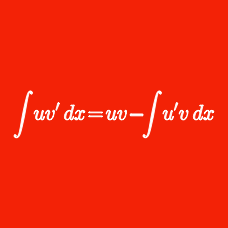Calculus

# Integration U-substitution - Trigonometric

Evaluate the integral $\displaystyle{ \int { \frac{10x}{\sqrt{3-2x-x^2}} dx }} ,$ using $C$ as the constant of integration.

Evaluate the integral $\displaystyle{ \int { \frac { 47 \sqrt{9-x^2} }{x^2 } dx } },$ using $C$ as the constant of integration.

Evaluate the integral $\displaystyle{ \int{\frac{15}{x^2\sqrt{x^2+4}}dx}},$ using $C$ as the constant of integration.

Evaluate the integral $\displaystyle{ 43\int { \cos^3 x dx} },$ using $C$ as the constant of integration.

Given $a>0 ,$ evaluate the integral $\displaystyle{ 41 \int { \frac{dx}{\sqrt{x^2 - a^2}} }},$ using $C$ as the constant of integration.

×